Orthogonal Polynomials

Orthogonal polynomials are classes of Polynomials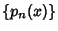over a rangewhich obey an Orthogonality relation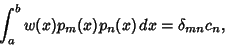(1)

where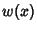is a Weighting Function andis the Kronecker Delta. If, then the Polynomials are not only orthogonal, but orthonormal.

Orthogonal polynomials have very useful properties in the solution of mathematical and physical problems. Just as Fourier Series provide a convenient method of expanding a periodic function in a series of linearly independent terms, orthogonal polynomials provide a natural way to solve, expand, and interpret solutions to many types of important Differential Equations. Orthogonal polynomials are especially easy to generate using Gram-Schmidt Orthonormalization. Abramowitz and Stegun (1972, pp. 774-775) give a table of common orthogonal polynomials.

 Type IntervalChebyshev Polynomial of the First Kind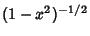Chebyshev Polynomial of the Second Kind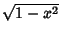Hermite Polynomial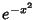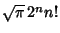Jacobi Polynomial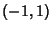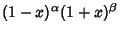Laguerre Polynomial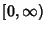1 Laguerre Polynomial (Associated)Legendre Polynomial1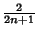Ultraspherical Polynomial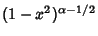In the above table, the normalization constant is the value of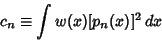(2)

and(3)

whereis a Gamma Function.

The Roots of orthogonal polynomials possess many rather surprising and useful properties. For instance, letbe the Roots of thewithand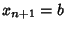. Then each interval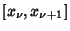for, 1, ...,contains exactly one Root of. Between two Roots ofthere is at least one Root of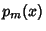for.

Letbe an arbitrary Real constant, then the Polynomial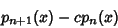(4)

has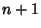distinct Real Roots. If(), these Roots lie in the interior of, with the exception of the greatest (least) Root which lies inonly for(5)

The following decomposition into partial fractions holds(6)

where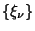are the Roots ofand(7)

Another interesting property is obtained by lettingbe the orthonormal set of Polynomials associated with the distributionon. Then the Convergentsof the Continued Fraction(8)

are given by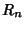(9)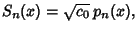(10)

where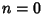, 1, ...and(11)

Furthermore, the Roots of the orthogonal polynomialsassociated with the distributionon the intervalare Real and distinct and are located in the interior of the interval.

See also Chebyshev Polynomial of the First Kind, Chebyshev Polynomial of the Second Kind, Gram-Schmidt Orthonormalization, Hermite Polynomial, Jacobi Polynomial, Krawtchouk Polynomial, Laguerre Polynomial, Legendre Polynomial, Orthogonal Functions, Spherical Harmonic, Ultraspherical Polynomial, Zernike Polynomial

References

Abramowitz, M. and Stegun, C. A. (Eds.). Orthogonal Polynomials.'' Ch. 22 in Handbook of Mathematical Functions with Formulas, Graphs, and Mathematical Tables, 9th printing. New York: Dover, pp. 771-802, 1972.

Arfken, G. Orthogonal Polynomials.'' Mathematical Methods for Physicists, 3rd ed. Orlando, FL: Academic Press, pp. 520-521, 1985.

Iyanaga, S. and Kawada, Y. (Eds.). Systems of Orthogonal Functions.'' Appendix A, Table 20 in Encyclopedic Dictionary of Mathematics. Cambridge, MA: MIT Press, p. 1477, 1980.

Nikiforov, A. F.; Uvarov, V. B.; and Suslov, S. S. Classical Orthogonal Polynomials of a Discrete Variable. New York: Springer-Verlag, 1992.

Sansone, G. Orthogonal Functions. New York: Dover, 1991.

Szegö, G. Orthogonal Polynomials, 4th ed. Providence, RI: Amer. Math. Soc., pp. 44-47 and 54-55, 1975.